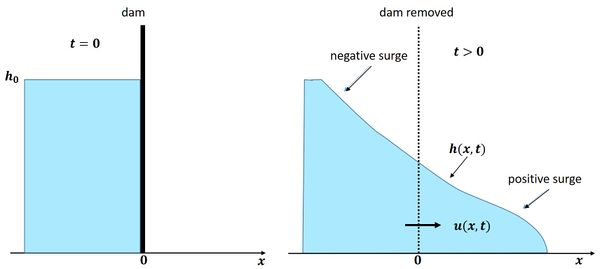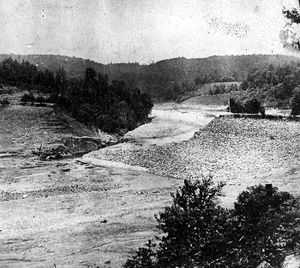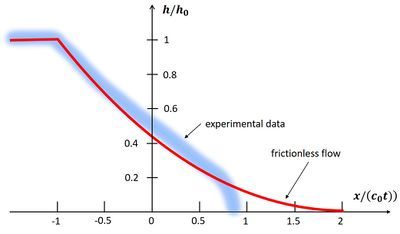# Dam break flow

This article discusses the often catastrophic flows that result from the failure of high dams that protect low-lying land. Dam break mechanisms are not dealt with; for this the reader is referred to the extensive literature existing on this subject, see for example Zhang et al. (2016)  and Almog et al. (2011) .

## IntroductionFig. 1. Sea dikes protecting low-lying polders in the Netherlands were breached during the extreme storm surge of 31 January 1953.

Dam failure can lead to disastrous situations. Most dam break tragedies are related to the collapse of reservoir dams in mountain rivers. The failure of sea dikes can also cause major disasters, although in this case the level difference is not as large. A dramatic example is the failure of more than fifty sea dikes in the Netherlands during the extreme storm surge of 1953, see figure 1. Many of these dikes protected land lying below the average sea level, while the storm surge level exceeded more than five meters. Rapid flooding killed more than two thousand people who were unable to flee in time to safe places. The most common dam failure mechanisms are related to overtopping and seepage (also called piping or internal erosion). In the case of the 1953 storm surge, overtopping and subsequent scour of the interior dike slope was the most important dike failure mechanism.Fig. 2. Left panel: Schematic representation of water retention behind a dam. Right panel: Positive downstream and negative upstream surges following instantaneous dam removal.

The consequences of dam failure have been studied for more than a century. It nevertheless remains a challenging topic due to the high non-linearity of the flood wave propagation. The problem can be tackled with numerical models, but rough estimates and insight into the tidal wave dynamics can be obtained with analytical solution methods.

## Frictionless dam-break flow: analytical solution

Analytical solutions relate to idealized situations as depicted in Fig. 2. The initial situation consists of an infinite reservoir with a water level that is $h_0$ higher than the horizontal ground level downstream of the dam. Dam break is simulated by instantaneous removal of the dam. This causes a positive surge in the positive $x$-direction and a negative surge in the negative $x$-direction. The floor is dry in front of the positive surge and the body of water behind the negative surge is undisturbed: water level =$h_0$ and current speed $u = 0$.Fig. 3. The earth-filled South Fork Dam on Lake Conemaugh (Pennsylvania, US) collapsed in 1889, killing 2,209 people in downstream villages.

The study of the dam break-flow was triggered by several dam failures in the 19th century, in particular the breach of the South Fork Dam in Pennsylvania (USA) in 1889, Fig. 3. Three years later Ritter  published an exact analytical solution for dam-break flow by assuming that frictional effects can be ignored. The derivation is given in box 1. According to this solution, the tip of the positive surge advances at high speed (supercritical flow) given by $u_f = 2 c_0$, where $c_0 = \sqrt {gh_0}$ and $g$ is the gravitational acceleration. The shape of the positive and negative wave is a concave-up parabola,

$h(x,t)= h_0, \; x\lt -c_0 t; \quad h(x,t)= \Large\frac{h_0}{9}\normalsize \; (2 - \Large\frac{x}{c_0 t}\normalsize)^2 , \, -c_0 t\lt x\lt 2c_0 t; \quad h(x,t)= 0, \, x\gt 2c_0 t , \qquad (1)$

see Fig. 4. According to Eq. (1) and Box 1, the discharge through the breach per unit width is given by

$q = \frac{8}{27} h_0 \sqrt{g h_0}. \qquad (2)$

Figure 4 also shows the shape of the dam break wave that was observed in laboratory experiments. For small values of $x / t$, the frictionless solution corresponds fairly well with the observations. However, the front zone is different: the shape is a bull nose, rather than a sharp edge. The front also advances more slowly; the speed of the front decreases with time and is closer to $u_f = c_0$ than to $u_f = 2 c_0$. The reason for this difference is the neglect of frictional effects that are important in the thin fluid layer near the front.

## Dam-break flow with frictionFig. 4. Wave profile after dam break. The red curve corresponds to the frictionless solution Eq. 1. The blue band represents data from different laboratory experiments performed at times $\small t \sim (40-80) \, \sqrt {h_0 / g}\normalsize$ after removal of the dam  . The frictionless solution only depends on $x/t$. Observed wave profiles also depend on time $t$ because of the decreasing wave tip speed.

When friction terms are included in the flow equations, there is no exact analytical solution. It is generally assumed that the frictionless flow equations are approximately valid in a short period after dam break and for small values of $x / (c_0 t)$. In the front zone, where the water depth is small, the momentum balance is dominated by frictional momentum dissipation. If the inertia terms $\partial u / \partial t + u \partial u / \partial x$ in the momentum equation are ignored, then the current in the front region is mainly determined by the balance of gravitational acceleration $g \partial h / \partial x$ and shear stress $\tau$, the latter term being proportional to the square of the flow velocity. With such models the shape of the wave front is given by $h(s) \propto s^n, \; n=1/2$, where $s$ is the distance measured from the wave front. The wave front speed $u_f$ decreases with time. At large times $t\gt \gt t_{\infty}$, the wave front speed varies approximately as $u_f \approx c_0 \sqrt{t_{\infty}/t}$ . Here, $t_{\infty} = \sqrt{h_0/g}/(3 c_D)$ and $c_D$ is the friction coefficient (value in the range $\approx (1-5)\, 10^{-3}$). A more elaborate model of the frictional boundary layer near the wave front was studied by Nielsen (2018) . According to this model, the wavefront is even more blunt, corresponding to $n = 1/4$, which agrees well with laboratory experiments. This model also reveals a strong downward velocity component at the wavefront, which can contribute to the dislodging of particles from the sediment bed . This may explain the high near-bed of suspended concentrations associated with propagating surges observed in the field, see also the article Tidal bore dynamics.

Data from numerous reservoir dam breaks that have occurred in the past have provided empirical formulas for the maximum discharge $Q_{max}$ through the breach . From this data it was deduced that the width of the breach is mainly related to the volume of water $V$ in the reservoir (volume above breach level). A reasonable estimate of the width is given by  $B \approx 0.3 V ^ {1/3}$. Empirical formulas for the maximum dam break discharge then yield  

$Q_{max} \approx 0.04 \sqrt{g} \, V^{0.37} h_0^{1.4} \approx 0.15 h_0 \, B \, \sqrt{g h_0} \, V^{0.04} h_0^{-0.1} , \qquad (3)$

or

$q_{max} \approx 0.22 h_0 \, \sqrt{g h_0} , \qquad (4)$

where in the approximation for the maximum discharge per unit width we have considered a large reservoir volume ($V \approx 10^7 m^3$) and a water depth $h_0 \approx 15 m$. The empirical estimate (4) is about 75% of the estimate (2) given by the frictionless flow solution.

## Numerical models

The analytical methods for describing dam-break flow provide a qualitative picture and some first-order estimates for the wave profile and wave speed. They may only be used in situations that can be represented schematically by a simple prismatic channel. In most actual field situations, the dam-break flow must be modeled numerically. Detailed simulation of the forward and backward wave fronts requires 3D models of the Boussinesq type, taking into account vertical fluid accelerations. To correctly model the strong temporal and spatial gradients of the surging wave, a fine computational grid is required, with resulting long computer times. See also Tidal bore dynamics.